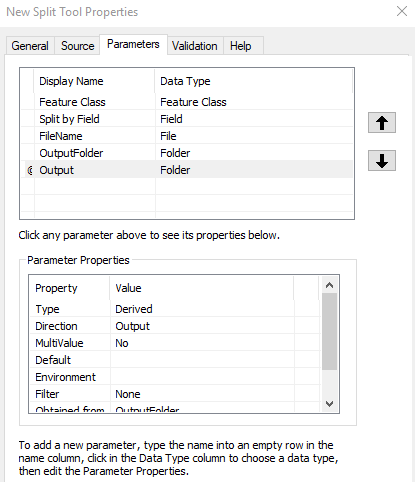# Error in using arcpy.SetParameterAsText

1155
5
03-22-2018 09:45 AMNew Contributor II

Hey All,

I am trying to create a derived output using a Python script. when I run the script, I keep getting the following error:

"RuntimeError: Object: Error in setting parameter as text". I have an output folder that is an input (outFolder). I basically want to use that same folder as an output once the script has run so that I can use it in a model.

My parameters are set up like this:

input_FC = arcpy.GetParameterAsText(1)
inField = arcpy.GetParameterAsText(2)
theFName = arcpy.GetParameterAsText(3)
outFolder = arcpy.GetParameterAsText(4)
output = arcpy.SetParameterAsText(5, outFolder)
script = sys.argv

I have tried several variations of SetParameterAsText, including:

outFolder = arcpy.SetParameterAsText(5, "outFolder")

and

arcpy.SetParameterAsText(5, outFolder)

My parameter named "output" is configured as such in the script tool properties:It is set to derived so that it will appear as an output in a model and obtained from the input parameter, outFolder (Named Feature Class in the parameter settings above). What am I doing wrong here?

1 Solution

Accepted SolutionsOccasional Contributor II

My first thought to solve this is have the first parameter as text set with an index of 0.

``````input_FC = arcpy.GetParameterAsText(0)
inField = arcpy.GetParameterAsText(1)
theFName = arcpy.GetParameterAsText(2)
outFolder = arcpy.GetParameterAsText(3)
output = arcpy.SetParameterAsText(4, outFolder)
script = sys.argv‍‍‍‍‍‍``````

See if that solves it.

5 RepliesOccasional Contributor II

My first thought to solve this is have the first parameter as text set with an index of 0.

``````input_FC = arcpy.GetParameterAsText(0)
inField = arcpy.GetParameterAsText(1)
theFName = arcpy.GetParameterAsText(2)
outFolder = arcpy.GetParameterAsText(3)
output = arcpy.SetParameterAsText(4, outFolder)
script = sys.argv‍‍‍‍‍‍``````

See if that solves it.byEsri Esteemed Contributor

You should start numbering with 0, not 1. Index number 5 does not exist.New Contributor II

Hey Guys- You are both absolutely correct. For whatever reason, I thought that arcpy.SetParameterAsText started its index at 1. This was the crux of my whole problem. It works beautifully now. Thanks so much.New Contributor

Hello Justin, i have the same problem now and i have to input it in my thesis defense next week, is it possible to see what the whole of your script looks like?

Thanks